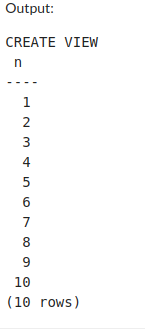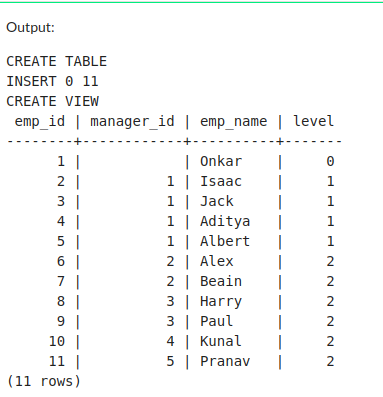Open in App
Not now

# PostgreSQL – Create Recursive Views

• Last Updated : 15 Sep, 2022

A view in PostgreSQL can be defined as a virtual table that is derived from underlying base tables or previously defined views. A view is nothing but a SQL statement that is stored in the database with an associated name. We have looked at what is the recursive query. To store the recursive query or to make a view out of a recursive query, PostgreSQL provides recursive views. So, recursive views are nothing but stored recursive queries.

In this article, we will learn about recursive views in PostgreSQL. We will introduce you to the concept of recursive views, show how recursive views are created, And most importantly we will also see how recursive views can be implemented in PostgreSQL.

### Creating PostgreSQL Recursive Views:

PostgreSQL provides two types of syntax for creating a recursive view.

Syntax:

CREATE RECURSIVE VIEW view_name(columns)

AS

SELECT …; — SQL query

Which is equivalent to:

CREATE VIEW view_name AS

WITH RECURSIVE view_name (column_names)

— recursive sql query

AS

SELECT …)

SELECT column_names

FROM view_name;

Let’s see some examples of recursive views.

Query:

```CREATE RECURSIVE VIEW fact(n, factorial) AS
(
SELECT 1 as n, 5 as factorial
union all
SELECT n+1, factorial*n FROM fact where n < 5
);
select * from fact;```

This code will create a view of the factorial of a natural number. which is the same as:

Query:

```CREATE VIEW fact AS
with recursive fact(n, factorial) as
(
SELECT 1 as n, 5 as factorial
union all
SELECT n+1, factorial*n FROM fact where n < 5
)
select * from fact;```

Both will give you the same output.

Here in this example, we are only considering n=5; We can print only the last row but here we can see how the iteration and calculation take place.

Output:

```CREATE VIEW
n | factorial
---+-----------
1 |         5
2 |         5
3 |        10
4 |        30
5 |       120
(5 rows)```

Let’s break down and understand what this code does:

• The very first line “CREATE VIEW fact AS ” includes keywords that indicate that we are creating a view “fact”.
• WITH RECURSIVE keywords indicate that it is a recursive query followed by a common table expression (CTE) and in parentheses, we have specified the list of columns that we are expecting in our result.
• Now there are two SELECT statements, the first one is non-recursive but the second one is calling “fact” recursively.
• And finally, the result set of two SELECT statements has been combined with the “UNION ALL” keyword.

Let’s take a look at another sample example, which is producing 1 to 10 numbers recursively.

Query:

```CREATE RECURSIVE VIEW tens(n) AS
(
SELECT 1 as n
UNION ALL
SELECT n+1 FROM tens
);
select * from tens limit 10;```

Output:Now let’s look at some real-life examples of recursive view, with the help of recursive view we can find the organizational hierarchy.

Step 1: Create a  table.

Query:

```create table emp(
emp_id int,
emp_name varchar(15),
manager_id int);```

Step 2: Insert data.

```INSERT INTO emp (
emp_id,
emp_name,
manager_id
)
VALUES
(1, 'Onkar', NULL),
(2, 'Isaac', 1),
(3, 'Jack', 1),
(5, 'Albert', 1),
(6, 'Alex', 2),
(7, 'Beain', 2),
(8, 'Harry', 3),
(9, 'Paul', 3),
(10, 'Kunal', 4),
(11, 'Pranav', 5);```

Step 3: This data represent hierarchy, e.g., Isaac is working under Onkar and Alex is working under Isaac. Thus Onkar is on top level. This example will give us results of which employee is working at which level.

Query:

```create recursive view subordinates(emp_id,
manager_id,emp_name, level) as
(
SELECT emp_id, manager_id, emp_name, 0 as level
FROM emp WHERE manager_id IS NULL
UNION ALL
SELECT e.emp_id, e.manager_id, e.emp_name, level+1
FROM emp e INNER JOIN subordinates s ON
s.emp_id = e.manager_id
);

SELECT * FROM subordinates;```

Output:My Personal Notes arrow_drop_up
Related Articles